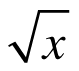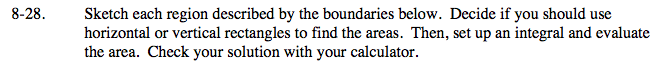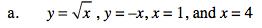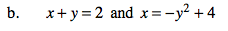### Home > CALC > Chapter 8 > Lesson 8.1.3 > Problem8-28

8-28.
1. Sketch each region described by the boundaries below. Decide if you should use horizontal or vertical rectangles to find the areas. Then, set up an integral and evaluate the area. Check your solution with your calculator. Homework Help ✎

1. y =, y = −x, x = 1, and x = 4

2. x + y = 2 and x = −y2 + 4Regarding horizontal or vertical rectangles... Is there a top function and a bottom function?
Or, is there a left-most function and a right-most function?
If it's the former, use vertical rectangles (and keep the equations written in y = ______________ form).
If it's the later, use horizontal rectangles (and keep the equations written as x = __________________ form).Regarding the graph of x = − y² + 4...
In order to visualize this graph, you should not have to rewrite the equation in y = ___________________ form.
You should know that it will look like a sleeping (sideways) parabola with vertex at x = 4.
The negative indicates that the parabola opens to the left.

Might as well be consistent and write the first function as x = ______________ as well.
Regarding horizontal or vertical rectangles...
Refer to the hint in part (a).